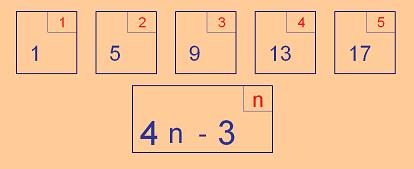#### You may also like### Diophantine N-tuples

Can you explain why a sequence of operations always gives you perfect squares?### DOTS Division

Take any pair of two digit numbers x=ab and y=cd where, without loss of generality, ab > cd . Form two 4 digit numbers r=abcd and s=cdab and calculate: {r^2 - s^2} /{x^2 - y^2}.### Sixational

The nth term of a sequence is given by the formula n^3 + 11n . Find the first four terms of the sequence given by this formula and the first term of the sequence which is bigger than one million. Prove that all terms of the sequence are divisible by 6.

# Interactive Number Patterns

##### Age 14 to 16Challenge LevelThis problem involves making a connection between the pattern in a sequence of numbers and the algebra that expresses the general term.

There are two parts to the problem and each has an Excel-based interactivity.

You can right-click and save to get your own copy of each resource: Interactive Number Patterns 1 and Interactive Number Patterns 2

Open the spreadsheet called Interactive Number Patterns 1 and experiment with the control buttons. You should discover that the red and blue numbers in the boxes, and also the algebra, can be made to show or not show. You should also discover that the numbers and the algebra can be changed.

Adjust the view size if necessary using Zoom on the View drop-down menu.

Challenge One has three stages :

• Hide the blue numbers in the boxes, change the algebra and the red numbers and check you can successfully predict the hidden blue values.

• Next hide the red numbers instead and repeat the challenge. Explain to a friend how you manage this. You could even work in pairs to set challenges for each other.

• Finally hide the algebra. Change it without showing it, then predict the formula. You have succeeded with this task when you can explain your method to someone else.

Challenge Two is similar but harder. Open the spreadsheet called Interactive Number Patterns 2. This interactivity works in the same way but now the algebra is quadratic :
• Predict the hidden blue

• Predict the hidden red, explaining your method

• Predict the hidden algebra, again explaining your method and especially why it works

The overall aim of this activity is to understand why our methods work - never be content to just remember a method.

And the "solutions" we would love to receive from you are your best ways of justifying this important method.

Even when we cannot immediately explain why a method is valid it is good to keep it in mind as something we very much want to know.